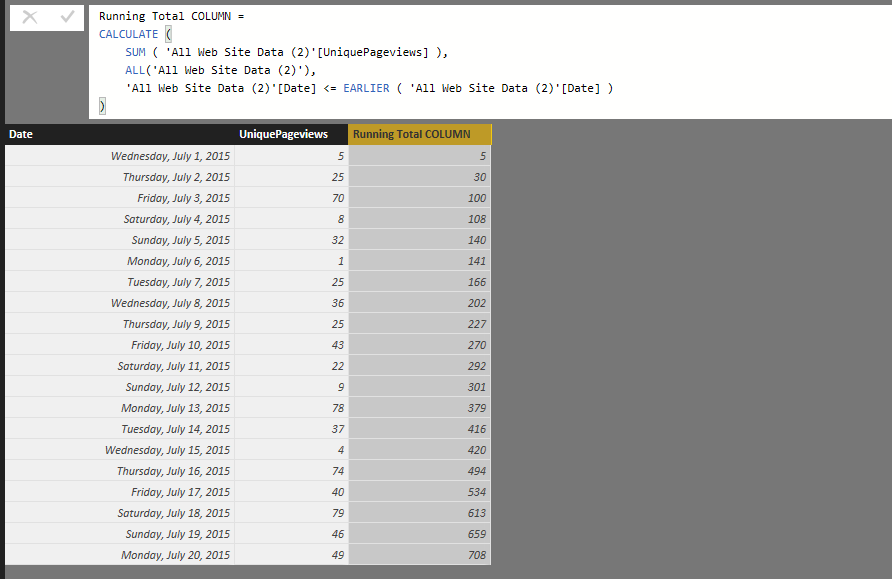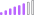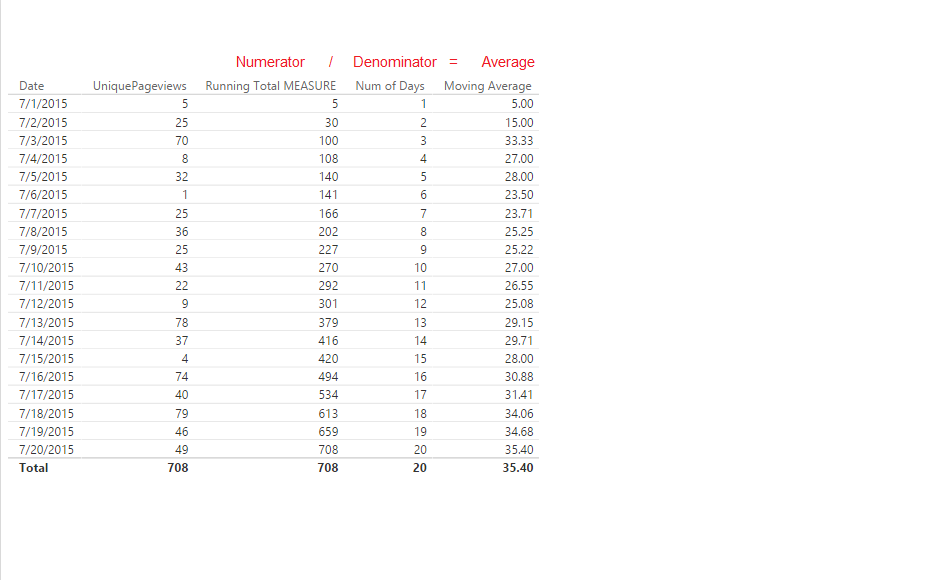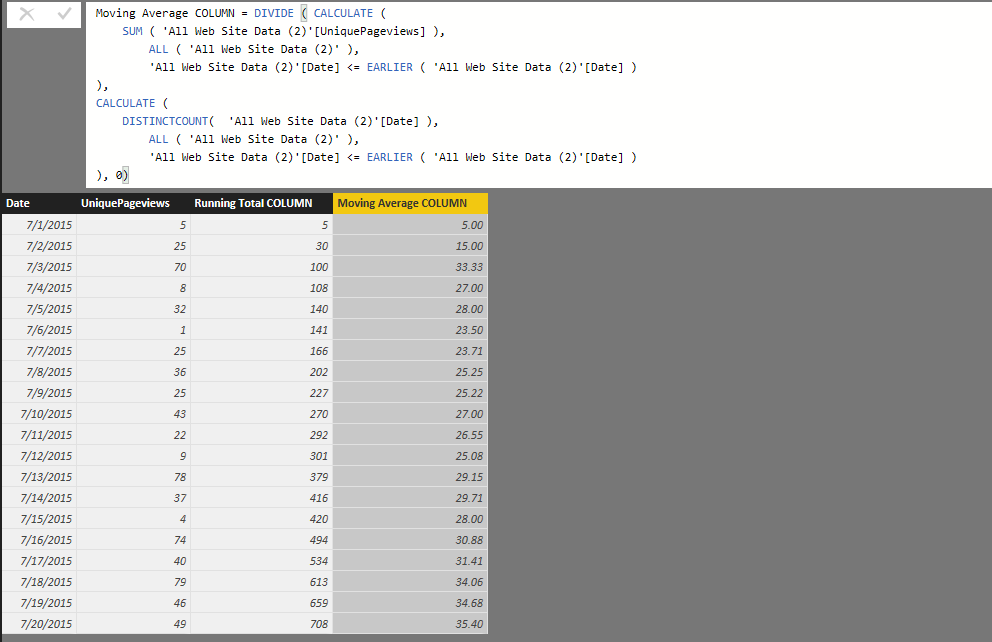cancel
Showing results for
Did you mean:

Fabric is Generally Available. Browse Fabric Presentations. Work towards your Fabric certification with the Cloud Skills Challenge.Post Prodigy

## Cumulative Total

Hi,

I'm at an absolute loss as to how to calculate a cumulative total. I've tried googling, reading the forums, following the documentation, decomposing the calculation, trying it as both a measure and a calc'd column; It always seems to refer me to the same number. So for example; March will be 10, April 12, but instead of showing me 10 for March and 22 for April, it shows me 10 for March and 12 for april.

For eg: https://gyazo.com/41bd333cedac290e6980772906ff0034 with a measure

I my Month column as a date column, I've tried using all kinds of features including, calc, sum, sumx, time based functions.

The commonly reccomended filter of [Date] <= MAX [Date] always returns an error. The Earlier function returns errors with concerns there isn't a function above it. Any help would be greatly appreciated; I've spent hours today trying to work this out and I get the feeling there is a difference in Pivottable dax and powerbi dax. I want to be able to shape and transform my data in powerbi, using dax in powerbi.

Any help would be greatly appreciated.

1 ACCEPTED SOLUTIONCommunity Champion

@ElliotP Sorry about the original post. It was from my phone and had typosOkay here is the formula for Running Total as a Calculated Column (prorerly formatted)

```Running Total COLUMN =
CALCULATE (
SUM ( 'All Web Site Data (2)'[UniquePageviews] ),
ALL ( 'All Web Site Data (2)' ),
'All Web Site Data (2)'[Date] <= EARLIER ( 'All Web Site Data (2)'[Date] )
)```

And as you can see it works!And here's the MEASURE formula

```Running Total MEASURE =
CALCULATE (
SUM ( 'All Web Site Data (2)'[UniquePageviews] ),
FILTER (
ALL ( 'All Web Site Data (2)' ),
'All Web Site Data (2)'[Date] <= MAX ( 'All Web Site Data (2)'[Date] )
)
)```

Which also works...79 REPLIES 79Post Patron

What is the workaround for it then?

Cumulative Scheduled Quantity = CALCULATE(SUM(F_PROJECT_PROGRESS_WORKMEN[Scheduled_QTY]),FILTER(ALL(D_DATE[Date_Key]),D_DATE[Date_Key]>=DATE(YEAR(TODAY()),MONTH(TODAY()),1) && D_DATE[Date_Key]<=[Today's Date]))

Cumulative Actual Quantity = CALCULATE(SUM(F_PROJECT_PROGRESS_WORKMEN[Actual_QTY]),FILTER(ALL(D_DATE[Date_Key]),D_DATE[Date_Key]>=DATE(YEAR(TODAY()),MONTH(TODAY()),1) && D_DATE[Date_Key]<=[Today's Date]))

These are the measures I created as I can't create Columns in Direct query mode. I didn't get the error you got though, unless I am misinformed on something here.Resolver I

1. Its Direct Query Mode (Live connection with SQL) not loaded the data in Power BI

2. Filter cannot be used in Direct Query Mode.

3. I have written this formula for measure not for column

My Question is:

I can't find a way to calculate a running total, without using FILTER. Filter is not supported in PowerBi direct query-mode.

All help is appreciated

Not supported in Direct Query ModeRunning Total in DAX = CALCULATE( SUM('Table'[QTY]),
FILTER(
ALLSelected('Table'),
'Table'[Date] <= MAX('Table'[Date])
)Regular Visitor

If i have a continuos data of the above kind, how will i calculate the culmative sum based on Month, Quarter and Year.

Thanks,
RaaghavanPost Prodigy

Thank you so much guys, I really appreciate it. It has been doing my head in.

Why do we use the filter feature for the measure but not the column? I checked and the measure formula works for a new column as well, but I'm curious as to explanation of the difference.

As well, I know this is going to be all the more complicated; But I'd also like to calculate a moving and trailing average. I'll have a try myself again, but if either @Sean or @Vvelarde knows the forumla off the top of their head, that would be greatly appreciated.

Thank you so much.Community Champion

@ElliotP Okay since you didn't mention how many Days or Month Average

Try this...

```Moving Average =
DIVIDE (
CALCULATE (
SUM ( 'All Web Site Data (2)'[UniquePageviews] ),
FILTER (
ALL ( 'All Web Site Data (2)' ),
'All Web Site Data (2)'[Date] <= MAX ( 'All Web Site Data (2)'[Date] )
)
),
CALCULATE (
DISTINCTCOUNT ( 'All Web Site Data (2)'[Date] ),
FILTER (
ALL ( 'All Web Site Data (2)' ),
'All Web Site Data (2)'[Date] <= MAX ( 'All Web Site Data (2)'[Date] )
)
),
0
)```

The Numerator is basically your Running Total Measure (so you actually can use the Measure name there) while

The Denominator is the number of days.

See picture to see how formula worksPost Patron

Hi,

My requirement is to display the cumulative sum for Actual and Scheduled Quantity from the start of the current month to the current day of the current month. Scheduled Quantity would be for the entire current month.

Current Month is Feb so X-axis would have days from 1 to 28. Y-Axis would show both cumulative sum of actual and scheduled quantity.

The actual and scheduled quantity is from the same table which also contains date key. The date key is common between this table and the date table.

I tried the measure -

Cumulative Scheduled Quantity = CALCULATE(SUM(F_PROJECT_PROGRESS_WORKMEN[Scheduled_QTY]),FILTER(ALL(D_DATE[Date_Key]),D_DATE[Date_Key]<=MAX(D_DATE[Date_Key]))) but I only get one bar at the end.Post PatronPost Prodigy

Thanks for the quick and explained reponse. I recieved the same thing; excep the Moving average values is the value for example for day 5 of 100, simply divided by 5 = 20. As opposed to being a running total divided by the number of days.

Something like

Day 1: 10

Day 2: 20

Day 3: 30

Day1avg: 10

Day2avg: 15

Day3avg: 20

I'll try and work it out, I'm trying to use the DATESBETWEEN function and some of the previousmonth and dateadd functions but I'm currently being told there are too few arguements (another issue).Post Prodigy

I've taken your function and added in the calculated column for Cumulative Quantity (running total); but now it gives me 1717 as it seems I'm not filtering it by the day.Post Prodigy

I've solved thef irst part using the EARLIER function

```Moving Average =
DIVIDE (
CALCULATE (
SUM ( [Cumulative Quantity1] ),
FILTER (
ALL ( 'All Web Site Data (2)' ),
'All Web Site Data (2)'[Date] <= EARLIER ( 'All Web Site Data (2)'[Date] )
)
),
CALCULATE (
DISTINCTCOUNT ( 'All Web Site Data (2)'[Date] ),
FILTER (
ALL ( 'All Web Site Data (2)' ),
'All Web Site Data (2)'[Date] <= EARLIER ( 'All Web Site Data (2)'[Date] )
)
),
0
)```

So it produces a literal moving average.

Now its a matter of creating a Measure to show YOY, Month on Month and day on day. This might be easier to break it into a few colums and calculate that way or use a Time Intelligent Function.Post Prodigy

I've created a Moving Average Measure and I'm now trying to create a column to produce a month on month moving average

```MovingaverageMeasure = CALCULATE([Movingaveragemonthmeasure],
DATESBETWEEN('All Web Site Data (2)'[Date],                    /*DATESBETWEEN function returns a table of days based on begin & end dates.*/
FIRSTDATE(PREVIOUSMONTH('All Web Site Data (2)'[Date])),   /*PREVIOUSMONTH gets all the days from the previous month. FIRSTDATE returns the first day of that month.*/
LASTDATE(DATEADD('All Web Site Data (2)'[Date],-1,MONTH))  /*DATEADD allows us to navigate a number of periods back in time. LASTDATE gets the last date.*/
)
)```

It's currently returnng the same numbers as my 'Moving Average' Column

----------------

Disregard, I forgot to send this a bit ago.Post Prodigy

Ok, I'm attempting to calculate the Running Total Column; we've pulled and shaped the data again and redone some things; When i use the code:

`Running Total Column = CALCULATE (DISTINCTCOUNT('All Web Site Data'[Date - Copy],DATESINPERIOD('All Web Site Data'[Date - Copy], LASTDATE('All Web Site Data'[Date - Copy]),-1,DAY )))`

I recieve the error "too many arguements were pased to DISTINTCOUNT function. The maximum argument count for the function is 1."

Then when I add a bracket and have this as my code;

`Running Total Column = CALCULATE (DISTINCTCOUNT('All Web Site Data'[Date - Copy]), DATESINPERIOD('All Web Site Data'[Date - Copy], LASTDATE('All Web Site Data'[Date - Copy]),-1,DAY ))`

I recieve "A circular dependency was detected: All Web Site Data[Running Total Column], All Web Site Data[Month on Month Return], All Web Site Data[Running Total Column]."

It seems we need to isolate the running total column from the other two columns mentioned?Post Prodigy

The running total colomn is the same as my Cumulative Quantity1 column:

https://gyazo.com/ddbe9a3af55579df7d1f9cfc03daf008

Now let me try something here....Post Prodigy

I just tried:

`Month on Month Total Sessions = Calculate(DISTINCTCOUNT('All Web Site Data'[Cumulative Quantity1]), DATESINPERIOD('All Web Site Data'[Date - Copy], LASTDATE('All Web Site Data'[Date - Copy]),-1, MONTH))`

And recieved a circular dependency bug.

Let me try using Sum instead of Distinctcount.

Returned Circular dependency. hmmmmPost Prodigy

I deleted the interferring column and created it as both a column and as a measure.

As a column it simply shows the same values as "Cumulative Quantity1" my already running total.

I tried adding the columns and the measures to graphs on my report page; yet when i add cumulative total it seems to mess up and shows tremendously high numbers in total on a bar graph, as in, it's high from the very beginning, as opposed to building and becoming bigger and bigger each month for example.

The same happens with my Moving average column.

I get the sense the graph issue may have something to do with the way the graph is interacting with the columns.Post Prodigy

I've created a measure;

```Month on Month Total SessionsMeasuree = CALCULATE([Cumulative Quantity1M],
DATESBETWEEN('All Web Site Data'[Date - Copy],                    /*DATESBETWEEN function returns a table of days based on begin & end dates.*/
FIRSTDATE(PREVIOUSMONTH('All Web Site Data'[Date - Copy])),   /*PREVIOUSMONTH gets all the days from the previous month. FIRSTDATE returns the first day of that month.*/
LASTDATE(DATEADD('All Web Site Data'[Date - Copy],-1,MONTH))  /*DATEADD allows us to navigate a number of periods back in time. LASTDATE gets the last date.*/
)
)```

But this occurs:

https://gyazo.com/3ecd0c362afd630dfa71661d0a7dd1cdCommunity Champion

Okay great! Yes as you said - literal moving average because you didn't specify time period 7 Day, 1 Month, 6 weeks, 3 months...

For those you have to create the corresponding Running total first (which would again be your numerator)

say 3 months and then use something like this for the denominator

`CALCULATE ( DISTINCTCOUNT(Calendar[Year-Month), DATESINPERIOD(CalendarTable[Date], LASTDATE(CalendarTable[Date]),-3,Month ) )`

Yes I should have mentioned the Moving Average formula I posted was a Measure!

Here's the Column...Announcements#### Power BI Monthly Update - November 2023

Check out the November 2023 Power BI update to learn about new features.#### Fabric Community News unified experience

Read the latest Fabric Community announcements, including updates on Power BI, Synapse, Data Factory and Data Activator.#### The largest Power BI and Fabric virtual conference

130+ sessions, 130+ speakers, Product managers, MVPs, and experts. All about Power BI and Fabric. Attend online or watch the recordings.Top Solution Authors
Top Kudoed Authors
Users online (3,788)